ISEE Middle Level Math : Quadrilaterals

Example Questions

1 2 11 12 13 14 15 16 17 19 Next →

Example Question #5 : How To Find The Area Of A Parallelogram

Find the area of a parallelogram with a base of 6 inches and a height of 9 inches.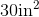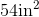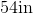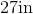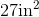Explanation:

To find the area of a parallelogram, we will use the following formula: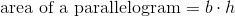where is the base and h is the height of the parallelogram.

Now, we know the base has a length of 6 inches. We also know it has a height of 9 inches.  Knowing this, we can substitute into the formula.  We get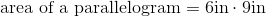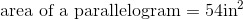Example Question #6 : How To Find The Area Of A Parallelogram

Find the area of the parallelogram with a base length of 6 and a height of 15.Explanation:

Write the area formula of a parallelogram.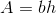Substitute the dimensions into the formula.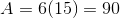The answer is:1 2 11 12 13 14 15 16 17 19 Next →